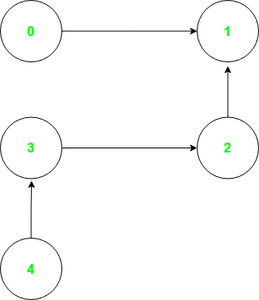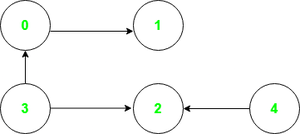# Smallest set of vertices to visit all nodes of the given Graph

• Difficulty Level : Medium
• Last Updated : 09 May, 2022

Given a directed acyclic graph of N nodes, the task is to find the smallest set of vertices from which the complete graph can be visited.

Examples:

Input: Graph in the image belowOutput: 0 4
Explanation: From vertex 0, the set of nodes that can be visited is {0 ,1}. Similarly, from vertex 4, {4, 3, 2} can be visited. Hence, the complete graph can be visited from the set {0, 4} which is of the minimum possible size.

Input: Graph in the image belowOutput: 3 4

Approach 1: The given problem can be solved using topological sorting to get the ordering of vertices such that for every directed edge from U to V, U comes before V. Below are the steps to follow:

• Sort the given array of vertices in topological order using Khan’s Algorithm.
• Maintain an array visited which keeps track of the visited vertices.
• Iterate the sorted array perform the following operations:
• If the current vertex is not visited, insert it into the required set.
• Visit all the nodes that are reachable from the inserted node using DFS traversal.

Below is the implementation of the above approach:

## Python3

 `# Python program of the above approach``from` `collections ``import` `defaultdict, deque` `class` `Solution:` `      ``# Function to perform DFS``    ``def` `dfs(``self``, node, vis, graph):` `        ``# add node to visited set``        ``vis.add(node)` `        ``for` `adj ``in` `graph[node]:``            ``if` `(adj ``not` `in` `vis):``                ``self``.dfs(adj, vis, graph)` `    ``def` `solve(``self``, edges):` `        ``graph ``=` `defaultdict(``list``)` `        ``# dictionary storing``        ``# indegrees of node``        ``indeg ``=` `defaultdict(``int``)` `        ``# array to store topological``        ``# sorting of the array``        ``topo_sort ``=` `[]``        ``vis ``=` `set``()` `        ``for` `(u, v) ``in` `edges:``            ``graph[u].append(v)` `            ``# count indegree of each node``            ``indeg[v] ``+``=` `1` `        ``qu ``=` `deque()` `        ``for` `u ``in` `graph:` `            ``# add to ququ ,if indegree``            ``# of node u is 0``            ``if``(indeg[u] ``=``=` `0``):``                ``qu.append(u)` `        ``# Run till queue is not empty``        ``while``(qu):` `            ``node ``=` `qu.popleft()` `            ``# add node to topo_sort``            ``topo_sort.append(node)` `            ``# traverse adj nodes``            ``for` `adj ``in` `graph[node]:` `                ``# decrement count of indegree``                ``# of each adj node by 1``                ``indeg[adj] ``-``=` `1` `                ``# if count becomes 0, then``                ``# add adj to qu``                ``if` `(indeg[adj] ``=``=` `0``):``                    ``qu.append(adj)` `        ``vis ``=` `set``()``        ``ans ``=` `[]` `        ``# Take each node from topo_sort``        ``for` `node ``in` `topo_sort:` `            ``# check if node is visited``            ``if` `(node ``not` `in` `vis):` `                ``vis.add(node)``                ``ans.append(node)` `                ``# Mark all the reachable``                ``# nodes as visited``                ``self``.dfs(node, vis, graph)` `        ``# finally return ans``        ``return` `(ans)`  `obj ``=` `Solution()``edges ``=` `[[``0``, ``1``], [``2``, ``1``], [``3``, ``2``], [``4``, ``3``]]` `ans ``=` `obj.solve(edges)``print``(``" "``.join(``str``(n) ``for` `n ``in` `ans))`
Output
`0 4`

Time Complexity: O(N)
Auxiliary Space: O(N)

Approach 2: The given problem can also be solved using the observation that vertices with in-degree 0 are the vertices that can not be reached from any other vertex. Hence, the idea is to find the indegree of each vertex and insert the vertices with in-degree 0 into the required set, as all the other vertices can be visited eventually.

Below is the implementation of the above approach:

## C++

 `// C++ program of the above approach``#include ``using` `namespace` `std;` `// Function to find smallest set``// of vertices from which the``// complete graph can be visited``vector<``int``> solve(vector>& edges)``{``    ``map<``int``, ``int``> graph;` `    ``// Dictionary storing``    ``// indegree of nodes``    ``map<``int``, ``int``> indeg;` `    ``for``(``auto` `dt : edges)``    ``{``        ``graph[dt] = dt;``        ` `        ``// Count indegree of``        ``// each node``        ``indeg[dt] += 1;``    ``}` `    ``vector<``int``> ans;``    ``for``(``auto` `it = graph.begin();``             ``it != graph.end(); ++it)``    ``{``        ` `        ``// Add to ans, if indegree``        ``// of node u is 0``        ``if` `(!indeg.count(it->first))``            ``ans.push_back(it->first);``    ``}` `    ``// Return Ans``    ``return` `ans;``}` `// Driver code``int` `main()``{``    ``vector> edges = { { 0, 1 }, { 2, 1 },``                                  ``{ 3, 2 }, { 4, 3 } };` `    ``vector<``int``> ans = solve(edges);``    ``for``(``auto` `dt : ans)``        ``cout << dt << ``" "``;` `    ``return` `0;``}` `// This code is contributed by rakeshsahni`

## Java

 `// Java program of the above approach``import` `java.util.*;` `class` `GFG{` `// Function to find smallest set``// of vertices from which the``// complete graph can be visited``static` `Vector solve(``int``[][] edges)``{``    ``HashMap graph = ``new` `HashMap();` `    ``// Dictionary storing``    ``// indegree of nodes``    ``HashMap indeg = ``new` `HashMap();` `    ``for``(``int` `dt[] : edges)``    ``{``        ``graph.put(dt[``0``], dt[``1``]);``        ` `        ``// Count indegree of``        ``// each node``        ``if``(indeg.containsKey(dt[``1``])) {``            ``indeg.put(dt[``1``], indeg.get(dt[``1``])+``1``);``        ``}``        ``else``            ``indeg.put(dt[``1``], ``1``);``    ``}` `    ``Vector ans = ``new` `Vector();``    ``for` `(Map.Entry it : graph.entrySet())``    ``{``        ` `        ``// Add to ans, if indegree``        ``// of node u is 0``        ``if` `(!indeg.containsKey(it.getKey()))``            ``ans.add(it.getKey());``    ``}` `    ``// Return Ans``    ``return` `ans;``}` `// Driver code``public` `static` `void` `main(String[] args)``{``    ``int``[][]edges = { { ``0``, ``1` `}, { ``2``, ``1` `},``                                  ``{ ``3``, ``2` `}, { ``4``, ``3` `} };` `    ``Vector ans = solve(edges);``    ``for``(``int` `dt : ans)``        ``System.out.print(dt+ ``" "``);` `}``}` `// This code is contributed by shikhasingrajput`

## Python3

 `# Python program of the above approach``from` `collections ``import` `defaultdict` `class` `Solution:``    ` `    ``# Function to find smallest set``    ``# of vertices from which the``    ``# complete graph can be visited``    ``def` `solve(``self` `, edges):` `        ``graph ``=` `defaultdict(``list``)` `        ``# dictionary storing``        ``# indegree of nodes``        ``indeg ``=` `defaultdict(``int``)` `        ``for` `(u,v) ``in` `edges:``            ``graph[u].append(v)` `            ``# count indegree of``            ``# each node``            ``indeg[v] ``+``=``1` `        ``ans ``=` `[]``        ``for` `u ``in` `graph:``            ` `            ``# add to ans, if indegree``            ``# of node u is 0``            ``if``(indeg[u] ``=``=` `0``):``                ``ans.append(u)` `        ``# Return Ans``        ``return` `(ans)``            `  `obj ``=` `Solution()``edges ``=` `[[``0``,``1``] , [``2``,``1``] , [``3``,``2``] , [``4``,``3``] ]` `ans``=` `obj.solve(edges)``print``(``" "``.join(``str``(n) ``for` `n ``in` `ans))`

## C#

 `// C# program of the above approach``using` `System;``using` `System.Collections.Generic;` `public` `class` `GFG {` `    ``// Function to find smallest set``    ``// of vertices from which the``    ``// complete graph can be visited``    ``static` `List<``int``> solve(``int``[, ] edges)``    ``{``        ``Dictionary<``int``, ``int``> graph``            ``= ``new` `Dictionary<``int``, ``int``>();` `        ``// Dictionary storing``        ``// indegree of nodes``        ``Dictionary<``int``, ``int``> indeg``            ``= ``new` `Dictionary<``int``, ``int``>();``        ``for` `(``int` `k = 0; k < edges.GetLength(0); k++) {``            ``int` `keys = edges[k, 0];``            ``int` `values = edges[k, 1];``            ``graph.Add(keys, values);` `            ``// Count indegree of``            ``// each node``            ``if` `(indeg.ContainsKey(values)) {``                ``indeg[values] += 1;``            ``}``            ``else``                ``indeg.Add(values, 1);``        ``}` `        ``List<``int``> ans = ``new` `List<``int``>();``        ``foreach``(KeyValuePair<``int``, ``int``> it ``in` `graph)``        ``{` `            ``// Add to ans, if indegree``            ``// of node u is 0``            ``if` `(!indeg.ContainsKey(it.Key))``                ``ans.Add(it.Key);``        ``}` `        ``// Return Ans``        ``return` `ans;``    ``}``    ``public` `static` `int``[] GetRow(``int``[, ] matrix, ``int` `row)``    ``{``        ``var` `rowLength = matrix.GetLength(1);``        ``var` `rowVector = ``new` `int``[rowLength];` `        ``for` `(``var` `i = 0; i < rowLength; i++)``            ``rowVector[i] = matrix[row, i];` `        ``return` `rowVector;``    ``}``  ` `    ``// Driver code``    ``public` `static` `void` `Main(String[] args)``    ``{``        ``int``[, ] edges``            ``= { { 0, 1 }, { 2, 1 }, { 3, 2 }, { 4, 3 } };` `        ``List<``int``> ans = solve(edges);``        ``foreach``(``int` `dt ``in` `ans) Console.Write(dt + ``" "``);``    ``}``}` `// This code is contributed by 29AjayKumar`
Output
`0 4`

Time Complexity: O(N)
Auxiliary Space: O(N)

My Personal Notes arrow_drop_up